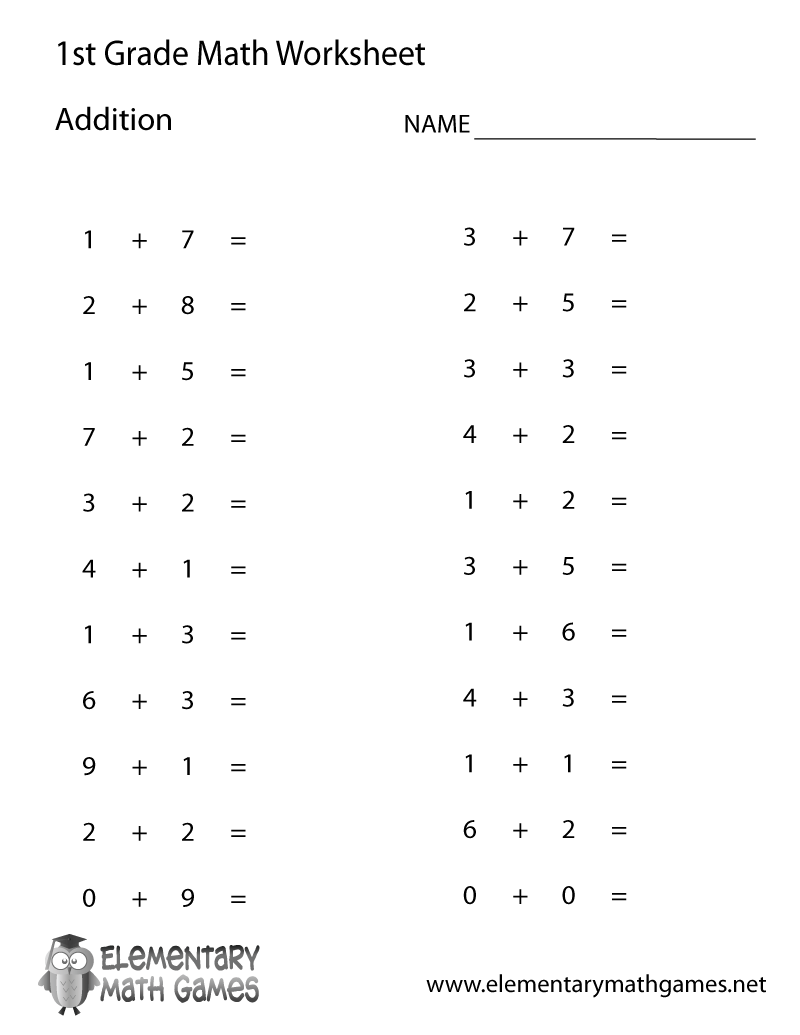## Learn and Practice How to Add Basic Numbers

This first grade math worksheet is a great way for kids to learn how to add. There are 22 simple math addition problems for first graders to solve.

Here are the two versions of this free first grade math worksheet: